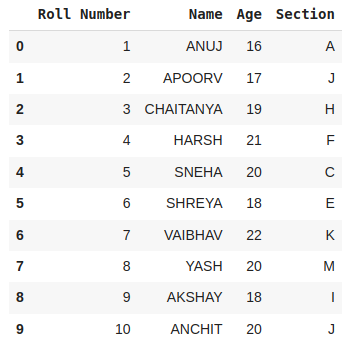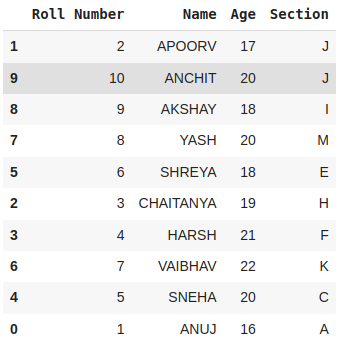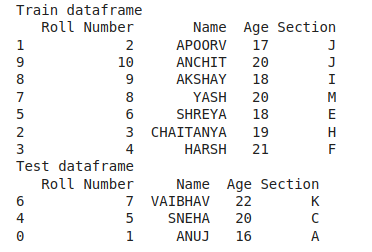Open in App
Not now

# How to split data into training and testing in Python without sklearn

• Difficulty Level : Easy
• Last Updated : 02 Feb, 2023

Here we will learn how to split a dataset into Train and Test sets in Python without using sklearn. The main concept that will be used here will be slicing. We can use the slicing functionalities to break the data into separate (train and test) parts. If we were to use sklearn this task is very easy but it can be a little tedious in case we are not allowed to use sklearn.

### Steps to split data into training and testing:

1. Create the Data Set or create a dataframe using Pandas.
2. Shuffle data frame using sample function of Pandas.
3. Select the ratio to split the data frame into test and train sets.
4. Split data frames into training and testing data frames using slicing.
5. Calculate total rows in the data frame using the shape function of Pandas.

Let’s implement these parts with an example.

## Python3

 `import` `pandas as pd` `# Creating sample dataset``df ``=` `pd.DataFrame({``    ``"Roll Number"``: [``1``, ``2``, ``3``, ``4``, ``5``, ``6``, ``7``, ``8``, ``9``, ``10``],``    ``"Name"``: [``" ANUJ"``, ``"APOORV"``, ``"CHAITANYA"``, ``"HARSH"``,``             ``" SNEHA"``, ``" SHREYA"``, ``"VAIBHAV"``, ``"YASH"``, ``"AKSHAY"``, ``"ANCHIT"``],``    ``"Age"``: [``16``, ``17``, ``19``, ``21``, ``20``, ``18``, ``22``, ``20``, ``18``, ``20``],``    ``"Section"``: [``'A'``, ``'J'``, ``'H'``, ``'F'``, ``'C'``, ``'E'``, ``'K'``, ``'M'``, ``'I'``, ``'J'``]``})``df`

Output:One of the challenges while splitting the data is that we would like to select rows randomly for the training as well as the training data. This functionality can be achieved by using the sample() method as shown below.

## Python3

 `# Shuffle dataframe using sample function``df ``=` `df.sample(frac``=``1``)``df`

Output:Shuffled data using the .sample() method

## Python3

 `# Select ratio``ratio ``=` `0.75` `total_rows ``=` `df.shape[``0``]``train_size ``=` `int``(total_rows``*``ratio)` `# Split data into test and train``train ``=` `df[``0``:train_size]``test ``=` `df[train_size:]`

Let’s print the training and testing part of the data.

## Python3

 `# print train set``print``(``"Train dataframe"``)``print``(train)` `# print test set``print``(``"Test dataframe"``)``print``(test)`

Output:Split data into train and testing

## Python3

 `train.shape, test.shape`

Output:

`((7, 4), (3, 4))`

My Personal Notes arrow_drop_up